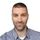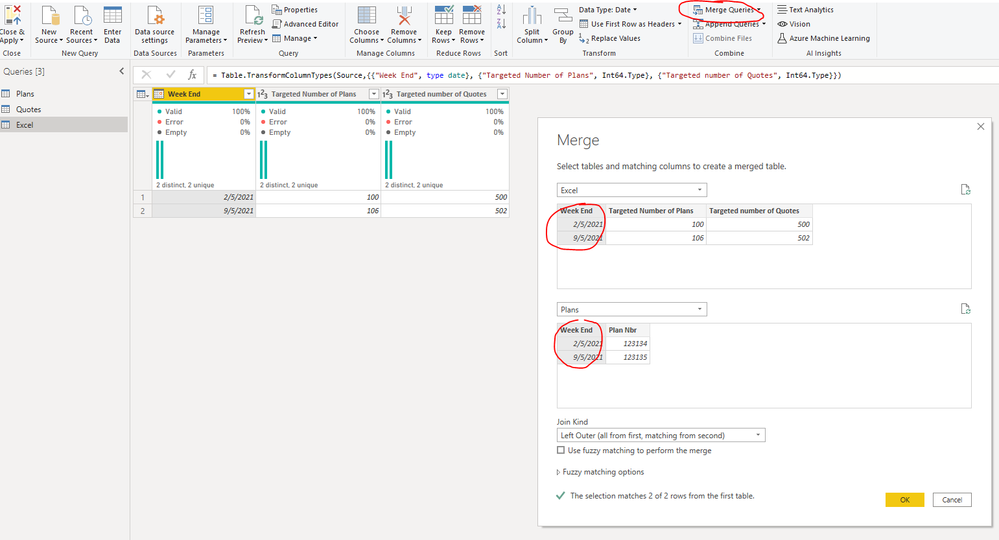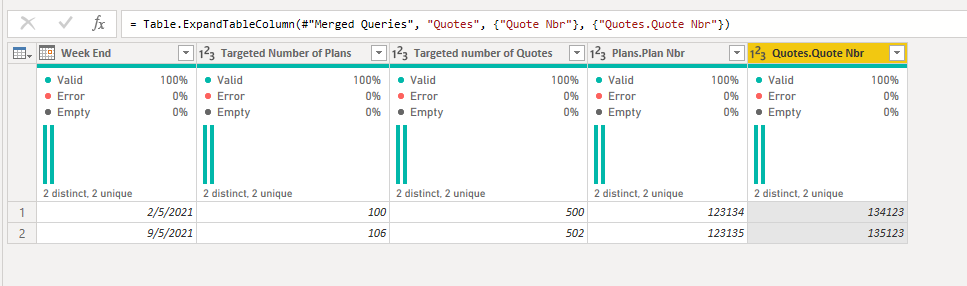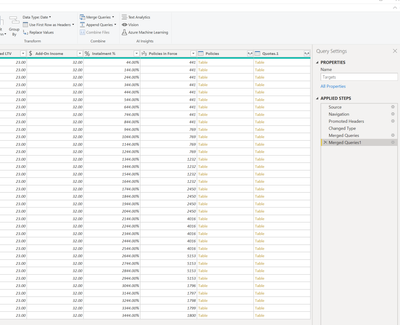cancel
Showing results for
Did you mean:Helper III

## formatting & merging 3 tables in matrix view

Hi -

I have 3 tables. Two are from SQL server so I don't think I can really alter it much. The other is an excel file. I need to connect the 3.

Table 1: SQL server looks like this:

 Week End Plan Nbr 2/05/2021 123134 9/05/2021 123135

**I then have to count distinct on Quote Nbr to get the number of Plans

Table 2: from SQL server

 Week End Quote Nbr 2/05/2021 123134 9/05/2021 123135

**I then have to count distinct on Quote Nbr to get the number of Quotes

Table 3: Excel file

 Week End Targeted Number of Plans Targeted number of Quotes 2/05/2021 100 500 9/05/2021 106 502 more future dates more forecasted data more forecasted data

Expected output for the most recent week (9/5/2021):

 Actual(count values from table 1 & 2) Target(table 3 valuesl) % Change Plans xx 106 x% Quotes xx 502 x%

I went into the model view and connected the tables on the week end column. I am now struggling to get the data in the format as above. It shows it like this:The problem is if I move the quotes to the column area - it doesn't count it up. I have it under values too but nothing seems to work how I need it to.

Would appreciate your help! so lost

1 ACCEPTED SOLUTIONMemorable Member

Hi @jnrezk ,

Did you try joining it in Power Query?
If there are 3 tables:
1) sql plans
2) sql quotes
3) excel - targets for plans and quotes
They all have week information. Use this week field to merge tables (as new query if needed) and you will have all fields in one table.
Start from table which will always have data.
Example: select Excel table, merge queries as new, merge with Plans table.
Then inside that new table, go again to merge queries as new, merget with Quotes table.
Expand needed columns (value, no need for week).Best regards,

Nemanja Andic

7 REPLIES 7Memorable Member

Hi @jnrezk , you accepted my answer as solution.
As @v-kelly-msft  provided in-depth details which meet your need, you should accept that answer as solution.

Cheers,
Nemanja AndicCommunity Support

Hi @jnrezk ,

First create a relationship between the tables from sql,like below:Then create a dim table as below:With three measures:

``````Target =
var _maxdate=MAXX(ALL(SQL1[Week End]),[Week End])
Return
SWITCH(SELECTEDVALUE('Table'[Column1]),
"Plans",CALCULATE(SUM(Excel[Targeted Number of Plans]),FILTER(ALL(Excel),'Excel'[Week End]=_maxdate)),
"Quotes",CALCULATE(SUM(Excel[Targeted number of Quotes]),FILTER(ALL(Excel),'Excel'[Week End]=_maxdate)))``````
``````Actual =
var _maxdate=MAXX(ALL(SQL1[Week End]),[Week End])
Return
SWITCH(SELECTEDVALUE('Table'[Column1]),
"Plans",CALCULATE(SUM('SQL1'[Plan Nbr]),FILTER(ALL('SQL1'),'SQL1'[Week End]=_maxdate)),
"Quotes",CALCULATE(SUM(SQL2[Quote Nbr]),FILTER(ALL(SQL2),'SQL2'[Week End]=_maxdate)))``````
``% change = DIVIDE('Table'[Actual]-'Table'[Target],'Table'[Actual])``

If you need calculated columns ,see below:

``````col_Actual =
var _maxdate=MAXX(ALL(SQL1[Week End]),[Week End])
Return
SWITCH('Table'[Column1],
"Plans",CALCULATE(SUM('SQL1'[Plan Nbr]),FILTER(ALL('SQL1'),'SQL1'[Week End]=_maxdate)),
"Quotes",CALCULATE(SUM(SQL2[Quote Nbr]),FILTER(ALL(SQL2),'SQL2'[Week End]=_maxdate)))``````
``````col_Target =
var _maxdate=MAXX(ALL(SQL1[Week End]),[Week End])
Return
SWITCH('Table'[Column1],
"Plans",CALCULATE(SUM(Excel[Targeted Number of Plans]),FILTER(ALL(Excel),'Excel'[Week End]=_maxdate)),
"Quotes",CALCULATE(SUM(Excel[Targeted number of Quotes]),FILTER(ALL(Excel),'Excel'[Week End]=_maxdate)))``````
``col_% change = DIVIDE('Table'[col_Actual]-'Table'[col_Target],'Table'[col_Actual])``

And you will see:For the related .pbix file,pls see attached.

Best Regards,
KellyHelper III

Thank you so much for your detailed response! I think it will work!! I have 2 follow up questions for now. I wanted a count distinct of the plan number but not sure if it worked this is how I did the actual code:

Actual =
var _maxdate=MAXX(ALL(Policies[WEEK_OF_YEAR_END_DATE]),[WEEK_OF_YEAR_END_DATE])
Return
SWITCH(SELECTEDVALUE('Summary'[Metric]),
"Plans",CALCULATE(DISTINCTCOUNT('Policies'[PLAN_NBR]),FILTER(ALL('Policies'), Policies[WEEK_OF_YEAR_END_DATE]=_maxdate)),
"Successful Quotes",CALCULATE(COUNTA(Quotes[Successful Quote Indicator]),FILTER(ALL(Quotes),'Quotes'[WEEK_OF_YEAR_END_DATE]=_maxdate)))

2) I also have a month field - how can I adjust the code so I can filter on either?

thank you again!Community Support

Hi  @jnrezk,

For question 1:

I think it will work.

For question 2:

If you also have a month filter,you could modify the variable as below:

``var _maxdate=Calculate(MAX([WEEK_OF_YEAR_END_DATE]),FILTER(ALL(Policies),[Month]=SELECTEDVALUE([MONTH])))``

If my method works,could you pls mark the reply as answered to let more people find it?

Best Regards,
KellyMemorable Member

Hi @jnrezk ,

Did you try joining it in Power Query?
If there are 3 tables:
1) sql plans
2) sql quotes
3) excel - targets for plans and quotes
They all have week information. Use this week field to merge tables (as new query if needed) and you will have all fields in one table.
Start from table which will always have data.
Example: select Excel table, merge queries as new, merge with Plans table.
Then inside that new table, go again to merge queries as new, merget with Quotes table.
Expand needed columns (value, no need for week).Best regards,

Nemanja AndicHelper III

Hi, i already connected on the date in the data model. I tried to do it as you said and it added these 2 things at the end. what do I do next was that right?Helper III

It is connecting to the server and I have input some SQL into the advanced box. The first table shows an ID - and i need to count distinct on how many of those IDs appear so it's not that number in the table that will be end result. Does that make sense? @nandic (responding here to your private message as it won't let me respond).